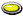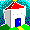MATH | Computer Science Student ResourcesMath Refresher: This brief overview covers useful high-school math you may have forgotten. Includes basic formulas for sinusoid and logarithmic functions, a discussion of log scales, and discrete probability. Includes a list of recommended inexpensive reference books. By William StallingsNumber Systems: Decimal, binary, hexadecimal, with a discussion of conversion from one system to another. By William Stallings.Pronunciation of mathematical expressions: The pronunciations of the most common mathematical expressions are given. A useful guide for everyone, especially ESL students and professionals.Queuing Analysis: A practical guide to an essential tool for computer scientists. By William Stallings.Math Help: Get Free Math help and Free online Math tutoring. Master basic and advanced Math concepts and also get help with your Math homework from best Online Math tutorTheoretical Computer Science Cheat Sheet: By Professor Steven Seiden. Ten pages of commonly used formulas and other useful information for computer scientists.Ask Dr. Math An excellent source of information on many math area. The emphasis is on high school math but college-level math is also covered.Applied Mathematics: Links to Math resources on the Web, organized by topic, plus by keyword search. The site also shows the level of mathematical background to read the materials.MathWorld: By the makers of Mathematica. Extensive, useful collection of information.Math Tables and Formulas: Tables featured include Trigonometric Identities, Derivatives, Indefinite Integrals, Common Integrals, and Binomial Coefficients and Formulas, among others. A search engine and links to other S.O.S. sites and the S.O.S. Mathematics Cyberboard (for posting questions) can also be found here.Math Reference Tables: Excellent collection of downloadable math tables.Prime Mathematics Encyclopedia: Large collection of entries on mathematical terms and concepts.Sage This computer algebra/computer mathematics package has the power and flexibility of Mathematica, Maple, or MATLAB. It is open source, free, and platform-independent. If you master one math package, this is the one to pick.Home Page of William Stallings